Courses

# Olympiad Test: Mirror Images - 2

## 20 Questions MCQ Test Mathematics Olympiad Class 7 | Olympiad Test: Mirror Images - 2

Description
This mock test of Olympiad Test: Mirror Images - 2 for Class 7 helps you for every Class 7 entrance exam. This contains 20 Multiple Choice Questions for Class 7 Olympiad Test: Mirror Images - 2 (mcq) to study with solutions a complete question bank. The solved questions answers in this Olympiad Test: Mirror Images - 2 quiz give you a good mix of easy questions and tough questions. Class 7 students definitely take this Olympiad Test: Mirror Images - 2 exercise for a better result in the exam. You can find other Olympiad Test: Mirror Images - 2 extra questions, long questions & short questions for Class 7 on EduRev as well by searching above.
QUESTION: 1

Solution:
QUESTION: 2

Solution:
QUESTION: 3

### Direction: In each of the following questions you are given a combination of alphabets and/or numbers followed by four alternatives (1), (2), (3) and (4). Q. Choose the alternative which closely resembles the mirror-image of the given combination.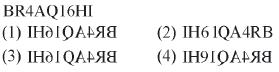Solution:
QUESTION: 4

Direction: In each of the following questions you are given a combination of alphabets and/or numbers followed by four alternatives (1), (2), (3) and (4).
Q. Choose the alternative which closely resembles the mirror-image of the given combination.

Solution:
QUESTION: 5

Direction: In each of the following questions you are given a combination of alphabets and/or numbers followed by four alternatives (1), (2), (3) and (4).
Q. Choose the alternative which closely resembles the mirror-image of the given combination.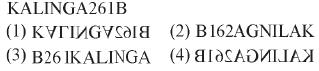Solution:
QUESTION: 6

Direction: In each of the following questions, choose the correct mirror image of the given image of the Fig.(X) from amongst the four alternatives (1), (2), (3) and (4) given along with it.
Q. Choose the correct mirror image of the given figure (X) from amongst the four alternatives.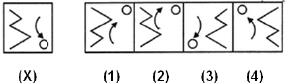Solution:
QUESTION: 7

Direction: In each of the following questions, choose the correct mirror image of the given image of the Fig.(X) from amongst the four alternatives (1), (2), (3) and (4) given along with it.
Q. Choose the correct mirror image of the given figure (X) from amongst the four alternatives.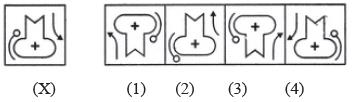Solution:
QUESTION: 8

Choose the correct mirror image of the given figure (X) from amongst the four alternatives.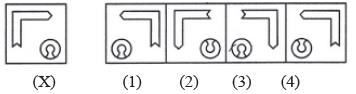Solution:
QUESTION: 9

Direction: In each of the following questions, choose the correct mirror image of the given image of the Fig.(X) from amongst the four alternatives (1), (2), (3) and (4) given along with it.
Q. Choose the correct mirror image of the given figure (X) from amongst the four alternatives.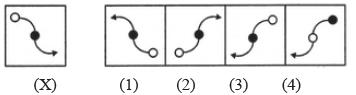Solution:
QUESTION: 10

Direction: In each of the following questions, choose the correct mirror image of the given image of the Fig.(X) from amongst the four alternatives (1), (2), (3) and (4) given along with it.
Q. Choose the correct mirror image of the given figure (X) from amongst the four alternatives.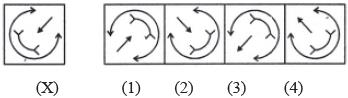Solution:
QUESTION: 11

Direction: In each of the following questions, choose the correct mirror image of the given image of the Fig.(X) from amongst the four alternatives (1), (2), (3) and (4) given along with it.
Choose the correct mirror image of the given figure (X) from amongst the four alternatives.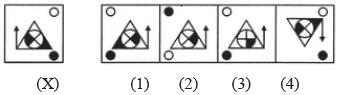Solution:
QUESTION: 12

Direction: In each of the following questions, choose the correct mirror image of the given image of the Fig.(X) from amongst the four alternatives (1), (2), (3) and (4) given along with it.
Q. Choose the correct mirror image of the given figure (X) from amongst the four alternatives.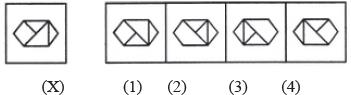Solution:
QUESTION: 13

Direction: In each of the following questions, choose the correct mirror image of the given image of the Fig.(X) from amongst the four alternatives (1), (2), (3) and (4) given along with it.
Q. Choose the correct mirror image of the given figure (X) from amongst the four alternatives.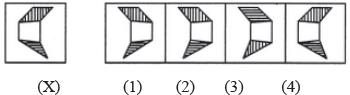Solution:
QUESTION: 14

Direction: In each of the following questions, choose the correct mirror image of the given image of the Fig.(X) from amongst the four alternatives (1), (2), (3) and (4) given along with it.
Q. Choose the correct mirror image of the given figure (X) from amongst the four alternatives.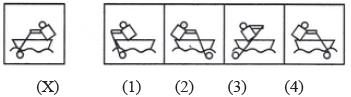Solution:
QUESTION: 15

Direction: In each of the following questions, choose the correct mirror image of the given image of the Fig.(X) from amongst the four alternatives (1), (2), (3) and (4) given along with it.
Q. Choose the correct mirror image of the given figure (X) from amongst the four alternatives.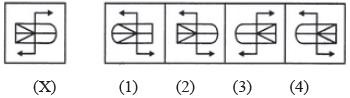Solution:
QUESTION: 16

Direction: In each of the following questions, choose the correct mirror image of the given image of the Fig.(X) from amongst the four alternatives (1), (2), (3) and (4) given along with it.
Q. Choose the correct mirror image of the given figure (X) from amongst the four alternatives.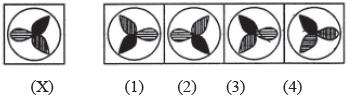Solution:
QUESTION: 17

Direction: In each of the following questions, choose the correct mirror image of the given image of the Fig.(X) from amongst the four alternatives (1), (2), (3) and (4) given along with it.
Q. Choose the correct mirror image of the given figure (X) from amongst the four alternatives.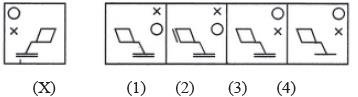Solution:
QUESTION: 18

Direction: In each of the following questions, choose the correct mirror image of the given image of the Fig.(X) from amongst the four alternatives (1), (2), (3) and (4) given along with it.
Q. Choose the correct mirror image of the given figure (X) from amongst the four alternatives.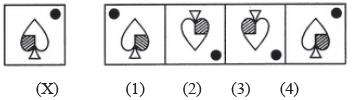Solution:
QUESTION: 19

Direction: In each of the following questions, choose the correct mirror image of the given image of the Fig.(X) from amongst the four alternatives (1), (2), (3) and (4) given along with it.
Q. Choose the correct mirror image of the given figure (X) from amongst the four alternatives.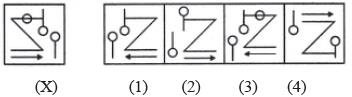Solution:
QUESTION: 20

Direction: In each of the following questions, choose the correct mirror image of the given image of the Fig.(X) from amongst the four alternatives (1), (2), (3) and (4) given along with it.
Q. Choose the correct mirror image of the given figure (X) from amongst the four alternatives.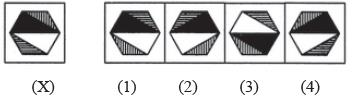Solution: